Math Courses / Course / Chapter

# Factors & Products

Shayna Sackett, Joseph Vigil
• Author
Shayna Sackett

Shayna has taught all subjects at the elementary level for over 15 years. She has a Bachelor’s degree in Early Childhood Education and a Master’s degree in Education Policy from University of Maryland.

• Instructor
Joseph Vigil

Joseph has a master's degree in literature as well as alternative teaching and ESL educator certifications. He has worked with middle school, high school, and college students in writing and language arts.

Learn the definition of factor pairs and about the product of two factors. Using examples, understand the difference between a factor and a multiple. Updated: 12/12/2021

Show

## Definition of Factor Pairs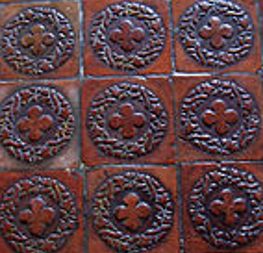There are many ways to count how many tiles there are in the picture, but since the tiles are arranged in an array, an equal number in each row, the most efficient way to count them is with multiplication. There are 3 rows with 3 tiles in each row. Each 3 represents a factor. To find the total, multiply the factor pairs 3 and 3, which equals 9 tiles in all. Factors are numbers multiplied to result in a total. Since a pair refers to two items, a factor pair is two factors.

### Product of Two Factors

When two numbers are multiplied, the answer they produce is called the product. In the tile example, 3 x 3 = 9. The product of 3 x 3 is 9 and 9 is divisible by 3.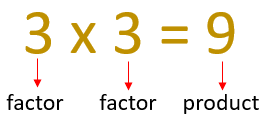The order of the factor pairs in a multiplication equation does not change the product. Factors can be multiplied forward and backward.

### Examples of Factor Pairs

Products can have more than one factor pair. For example, the number 6 has two factor pairs because 6 can be composed in different ways. The following arrays show various ways to make 6. The numbers of rows and columns are factors.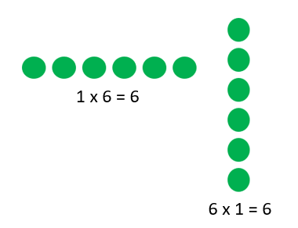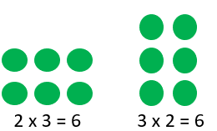The factors of 6 can be expressed in a list as 1, 2, 3, and 6 or as pairs 1 and 6 and 2 and 3. Since multiplication is commutative, the same forward and backward, it is not necessary to repeat factor pairs in reverse order. If 2 and 3 are a factor pair of 6, it is assumed that 3 and 2 are also a factor pair of 6.

Another number with more than one factor pair is 24. To find factor pairs for 24, think about the ways 24 can be divided evenly without remainders.

{eq}24 \div 6 = 4 {/eq} and {eq}24 \div 4 = 6 {/eq}

{eq}24 \div 2 = 12 {/eq} and {eq}24 \div 12 = 2 {/eq}

{eq}24 \div 3 = 8 {/eq} and {eq}24 \div 8 = 3 {/eq}

{eq}24 \div 1 = 24 {/eq} and {eq}24 \div 24 = 1 {/eq}

So, the factor pairs for 24 are 1 and 24, 2 and 12, 6 and 4, and 3 and 8. Or, listed out the factors of 24 are 1, 2, 3, 4, 6, 8, and 12.

Here are a few more factor pairs.

15: 1 and 15, 3 and 5

20: 1 and 20, 2 and 10, 4 and 5

27: 1 and 27, 3 and 9

## Definition of a Factor Pair

Let's say a mother in your neighborhood hires you to babysit for \$75 a night, and you can babysit four nights of the week. Of course, to figure out your weekly income, we would multiply the nightly income by the number of nights you can work:

75 * 4 = 300

You'll make \$300 in one week! Not bad.

Factors are the numbers you multiply together. In this multiplication sentence, 75 and 4 are the factors. 300 is the product, which is the answer we get when we multiply two factors together.

So, in this multiplication sequence, 3 * 4 = 12, 3 and 4 are the factors, and 12 is the product.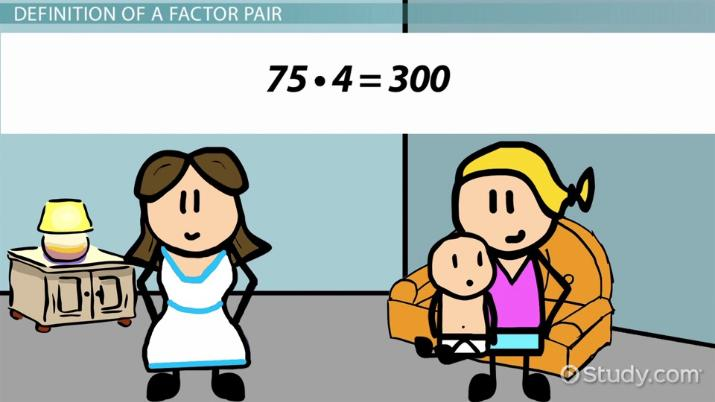An error occurred trying to load this video.

Try refreshing the page, or contact customer support.

Coming up next: 52 as a Product of Prime Factors: Steps & How-to

### You're on a roll. Keep up the good work!

Replay
Your next lesson will play in 10 seconds
• 0:05 Definition of a Factor Pair
• 0:46 Pairing Up
• 1:37 Examples
• 2:24 Zero - A Special Case
• 2:50 Lesson Summary
Save Save

Want to watch this again later?

Timeline
Autoplay
Autoplay
Speed Speed

## Pairing Up

Factor pairs are pairs of factors we multiply together to get a certain product. Let's go back to our second multiplication sentence: 3 * 4 = 12.

4 and 3 is one factor pair that can give us a product of 12. But we can also write the following multiplication sentences that give a product of 12:

• 1 * 12 = 12
• 2 * 6 = 12

So, the factor pairs that will give us a product of 12 are 1 and 12, 2 and 6, and 3 and 4.

Generally speaking, the larger a number is, the more factor pairs it will have. This isn't true for prime numbers because their only factor pairs are 1 and the number itself. For example, 937 is a large number, but it's also a prime number. So, its only factor pair is 1 and 937.

## Examples

Let's determine the factor pairs for 40. We can write the following multiplication sentences to get a product of 40:

• 1 * 40 = 40
• 2 * 20 = 40
• 4 * 10 = 40
• 5 * 8 = 40

So, 40's factor pairs are 1 and 40; 2 and 20; 4 and 10; 5 and 8.

To unlock this lesson you must be a Study.com Member.

Video Transcript

## Definition of a Factor Pair

Let's say a mother in your neighborhood hires you to babysit for \$75 a night, and you can babysit four nights of the week. Of course, to figure out your weekly income, we would multiply the nightly income by the number of nights you can work:

75 * 4 = 300

You'll make \$300 in one week! Not bad.

Factors are the numbers you multiply together. In this multiplication sentence, 75 and 4 are the factors. 300 is the product, which is the answer we get when we multiply two factors together.

So, in this multiplication sequence, 3 * 4 = 12, 3 and 4 are the factors, and 12 is the product.

## Pairing Up

Factor pairs are pairs of factors we multiply together to get a certain product. Let's go back to our second multiplication sentence: 3 * 4 = 12.

4 and 3 is one factor pair that can give us a product of 12. But we can also write the following multiplication sentences that give a product of 12:

• 1 * 12 = 12
• 2 * 6 = 12

So, the factor pairs that will give us a product of 12 are 1 and 12, 2 and 6, and 3 and 4.

Generally speaking, the larger a number is, the more factor pairs it will have. This isn't true for prime numbers because their only factor pairs are 1 and the number itself. For example, 937 is a large number, but it's also a prime number. So, its only factor pair is 1 and 937.

## Examples

Let's determine the factor pairs for 40. We can write the following multiplication sentences to get a product of 40:

• 1 * 40 = 40
• 2 * 20 = 40
• 4 * 10 = 40
• 5 * 8 = 40

So, 40's factor pairs are 1 and 40; 2 and 20; 4 and 10; 5 and 8.

To unlock this lesson you must be a Study.com Member.

#### What are factor pairs of 24?

There are several factors of 24. The factor pairs are 1 and 24, 2 and 12, 3 and 4, and 4 and 6.

#### What are the factor pairs of 12?

The factor pairs of 12 are 1 and 12, 2 and 6, and 3 and 4. 12 can be divided by any of these factors without a remainder.

#### What is a factor pair for 2?

2 has only one factor pair- 1 and 2. Since 2 has no other factor pairs, it is a prime number.

#### What are factors and factor pairs?

Factors are numbers that are multiplied together to equal a product. Factor pairs are the two numbers that are multiplied.

#### How do you find the factor pairs?

You can find factor pairs by creating an array for the total. The number of rows and columns are a factor pair. You can also use division to find factor pairs. When a number is divided by another, the divisor and quotient are factors, as long as the remainder is 0.

### Register to view this lesson

Are you a student or a teacher?

Back

### Resources created by teachers for teachers

Over 30,000 video lessons & teaching resources‐all in one place.Video lessonsQuizzes & WorksheetsClassroom IntegrationLesson Plans

I would definitely recommend Study.com to my colleagues. It’s like a teacher waved a magic wand and did the work for me. I feel like it’s a lifeline.

Jennifer B.
TeacherCreate an account to start this course today
Used by over 30 million students worldwide